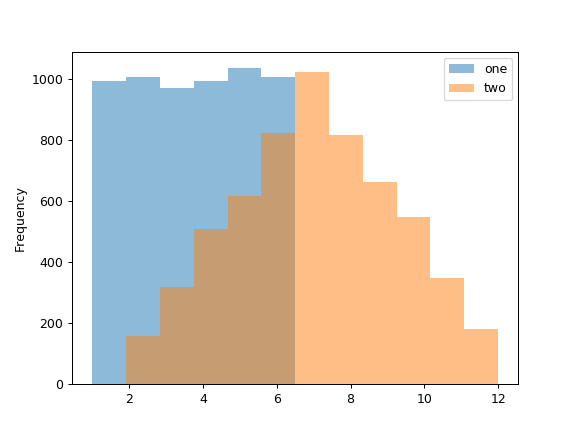/pandas 0.23

# pandas.DataFrame.plot.hist

`DataFrame.plot.hist(by=None, bins=10, **kwds)` [source]

Draw one histogram of the DataFrame’s columns.

A histogram is a representation of the distribution of data. This function groups the values of all given Series in the DataFrame into bins and draws all bins in one `matplotlib.axes.Axes`. This is useful when the DataFrame’s Series are in a similar scale.

Parameters: by : str or sequence, optional Column in the DataFrame to group by. bins : int, default 10 Number of histogram bins to be used. **kwds Additional keyword arguments are documented in `pandas.DataFrame.plot()`. `axes : matplotlib.AxesSubplot histogram.`

See also

`DataFrame.hist`
Draw histograms per DataFrame’s Series.
`Series.hist`
Draw a histogram with Series’ data.

#### Examples

When we draw a dice 6000 times, we expect to get each value around 1000 times. But when we draw two dices and sum the result, the distribution is going to be quite different. A histogram illustrates those distributions.

```>>> df = pd.DataFrame(
...     np.random.randint(1, 7, 6000),
...     columns = ['one'])
>>> df['two'] = df['one'] + np.random.randint(1, 7, 6000)
>>> ax = df.plot.hist(bins=12, alpha=0.5)
```© 2008–2012, AQR Capital Management, LLC, Lambda Foundry, Inc. and PyData Development Team
Licensed under the 3-clause BSD License.
http://pandas.pydata.org/pandas-docs/version/0.23.4/generated/pandas.DataFrame.plot.hist.html